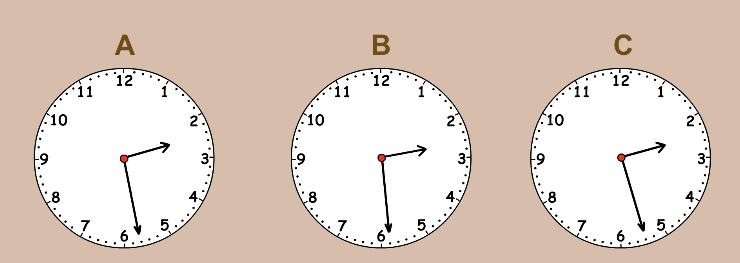ClockWork 8
Set 8 (Convert hours & minutes, as well as recognize expressions like, "Which clock shows ten minutes 'til...", when shown an analog clock.)
From Mr. Anker Tests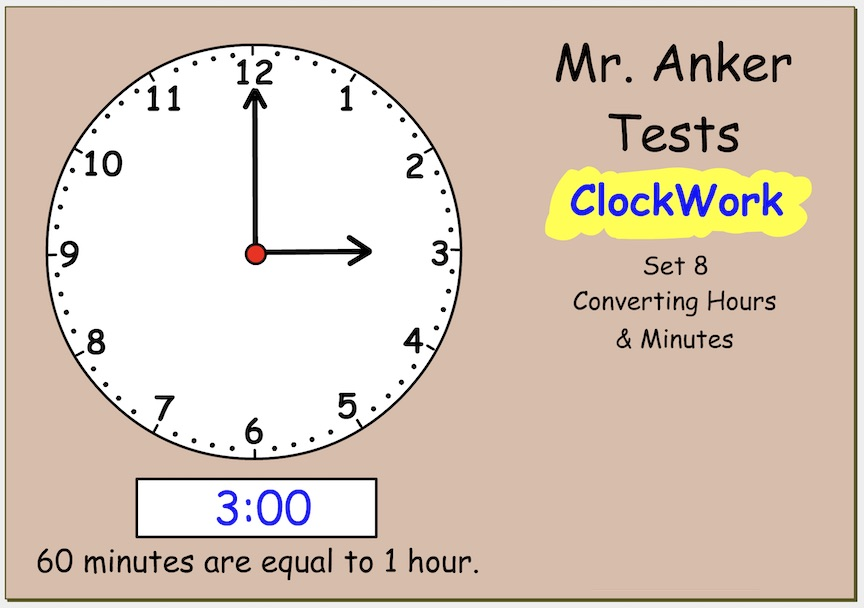1. How many minutes are there in one hour?
1 point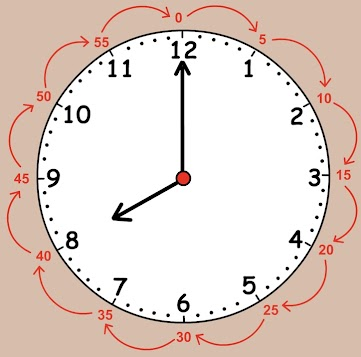2. How many minutes are there in 1/2 hour? *
1 point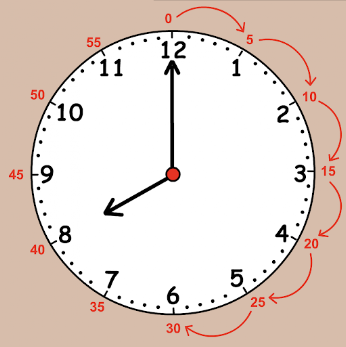3. How many minutes are there in two hours? *
1 point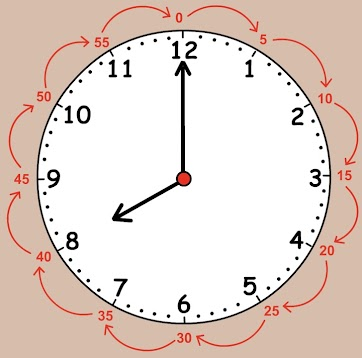4. How many minutes are there in three hours? *
1 point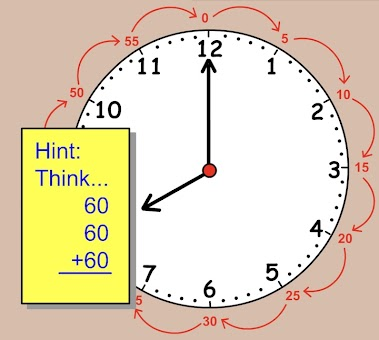5. How many hours are equal to 120 minutes? *
1 point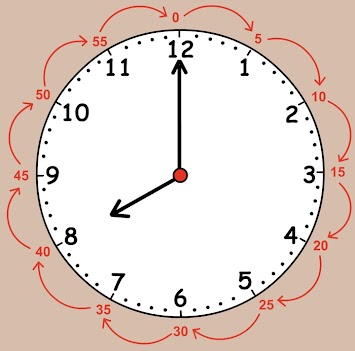6. Which of the clocks below shows the time, "seven minutes before nine"? *
1 point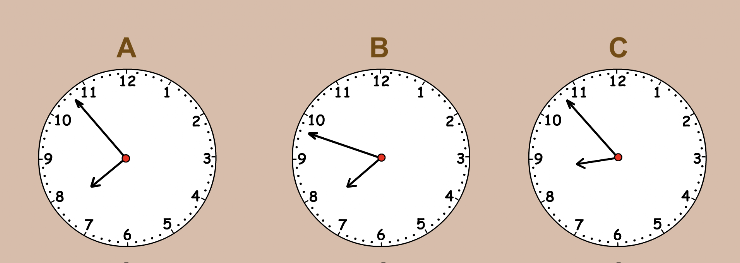7. Which of the clocks below shows the time, "six minutes after ten"? *
1 point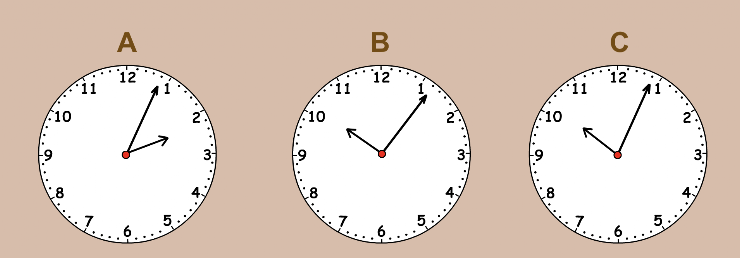8. Which of the clocks below shows the time, "twenty minutes 'til ten"? *
1 point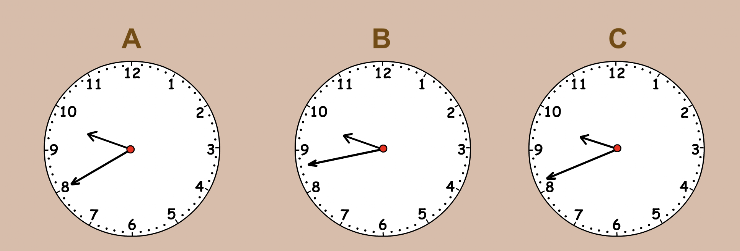9. Which of the clocks below shows a time that is closest to one o'clock? *
1 point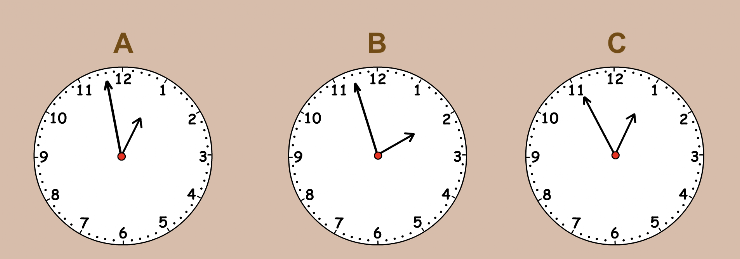10. Which of the clocks below will show the time, three o'clock, in thirty-one minutes? *
1 point